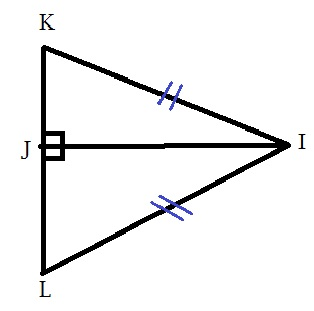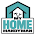## Tuesday, 17 December 2019

### Q1. In each pair of triangles in the following figure, parts bearing identical marks are congruent. State the Test and Correspondence of vertices by triangles in each pair of congruent:

(i) The triangles are congruent by SAS Test under correspondence
XW️Z↔️ YZW
(Here WZ is common side, and Z is 90° so its SAS Test)(ii)  The triangles are congruent by Hypotenuse side Test under the correspondence
KJ️I ↔️ LJI

(iii) The triangles are congruent by SSS Test under correspondence
HE️G↔️ FGE

(iv) The triangles are congruent by ASA Test under correspondence
SMA ↔️ OPT.(v) The triangles are congruent by SAA Test under the correspondence MT️N↔️ STN

#### 1 comment:

1.Useful content.